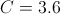Example Questions

Example Question #21 : How To Find The Length Of The Hypotenuse Of A Right Triangle : Pythagorean Theorem

A right triangle has legs of 15m and 20m. What is the length of the hypotenuse?

40m

35m

30m

45m

25m

25m

Explanation:

The Pythagorean theorem is a2 + b2 = c2, where a and b are legs of the right triangle, and c is the hypotenuse.

(15)2 + (20)2 = c2 so c2 = 625.  Take the square root to get c = 25m

Example Question #31 : Triangles

Paul leaves his home and jogs 3 miles due north and 4 miles due west. If Paul could walk a straight line from his current position back to his house, how far, in miles, is Paul from home?

7

√14

4

5

25

5

Explanation:

By using the Pythagorean Theorem, we can solve for the distance “as the crow flies” from Paul to his home:

32 + 42 = x2

9 + 16 = x2

25 = x2

5 = x

Example Question #22 : How To Find The Length Of The Hypotenuse Of A Right Triangle : Pythagorean Theorem

Given a right triangle where the two legs have lengths of 3 and 4 respectively, what is the length of the hypotenuse?

25

4

9

3

5

5

Explanation:

The hypotenuse can be found using Pythagorean Theorem, which is a+ b= c2, so we plug in a = 3 and b = 4 to get c.

c2  =25, so c = 5

Example Question #11 : How To Find The Length Of The Hypotenuse Of A Right Triangle : Pythagorean Theorem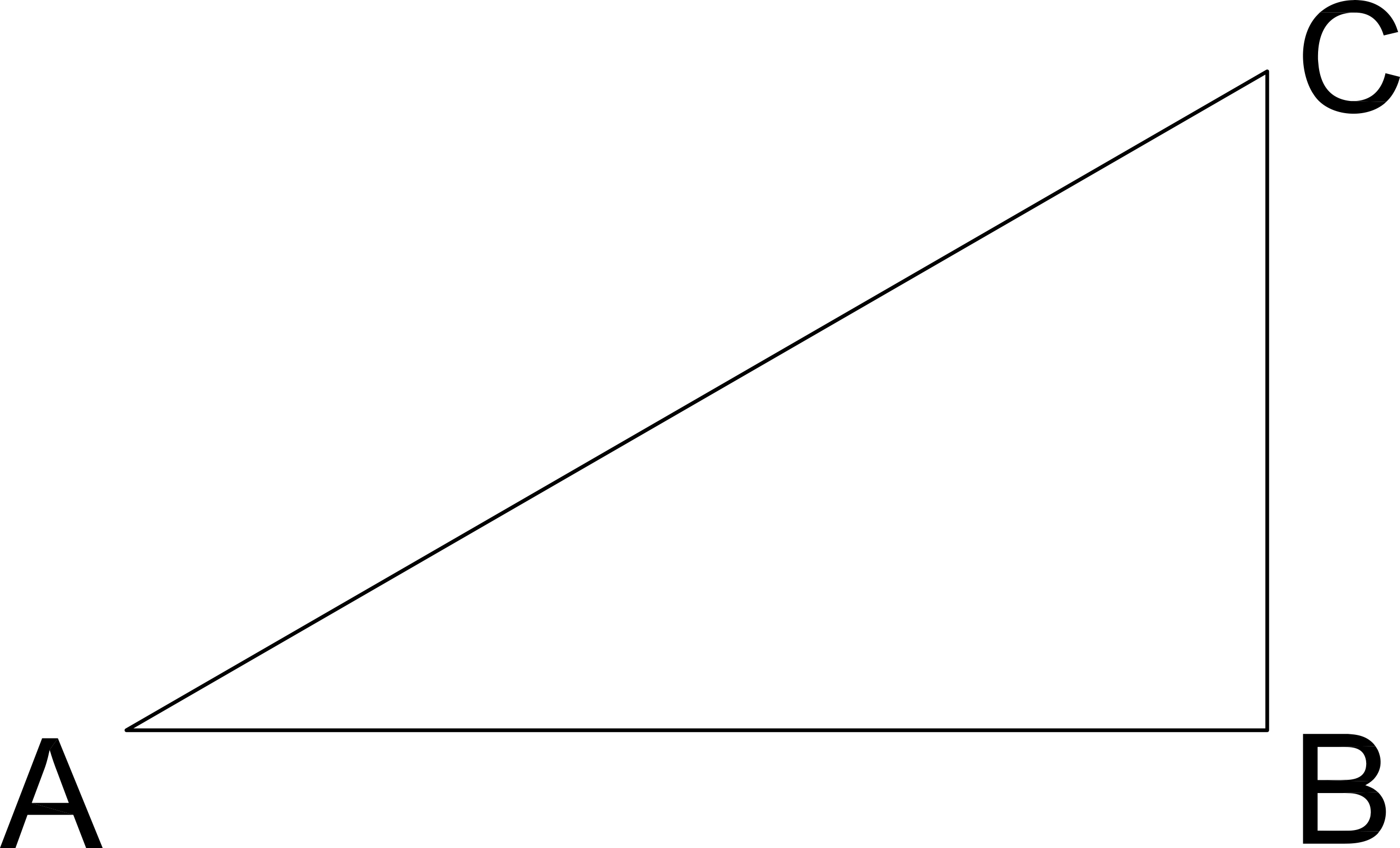Length AB = 4

Length BC = 3

If a similar triangle has a hypotenuse length of 25, what are the lengths of its two legs?

20 and 25

3 and 4

15 and 25

5 and 25

15 and 20

15 and 20

Explanation:

Similar triangles are in proportion.

Use Pythagorean Theorem to solve for AC:

Pythagorean Theorem:  AB2 + BC2 = AC2

42 + 32 = AC2

16 + 9 = AC2

25 = AC2

AC = 5

If the similar triangle's hypotenuse is 25, then the proportion of the sides is AC/25 or  5/25 or 1/5.

Two legs then are 5 times longer than AB or BC:

5 * (AB) = 5 * (4) = 20

5 * (BC) = 5 * (3) = 15

Example Question #61 : Sat Mathematics

If the base of a right triangle is 5 cm long and the height of the triangle is 7 cm longer than the base, what is the length of the third side of the triangle in cm?Explanation:

Find the height of the triangle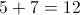Use the Pythagorean Theorem to solve for the length of the third side, or hypotenuse.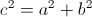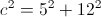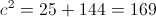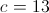Example Question #21 : How To Find The Length Of The Hypotenuse Of A Right Triangle : Pythagorean Theorem

Given the right triangle in the diagram, what is the length of the hypotenuse?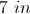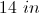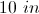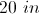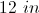Explanation:

To find the length of the hypotenuse use the Pythagorean Theorem: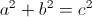Where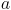andare the legs of the triangle, and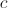is the hypotenuse.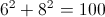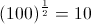The hypotenuse is 10 inches long.

Example Question #21 : How To Find The Length Of The Hypotenuse Of A Right Triangle : Pythagorean Theorem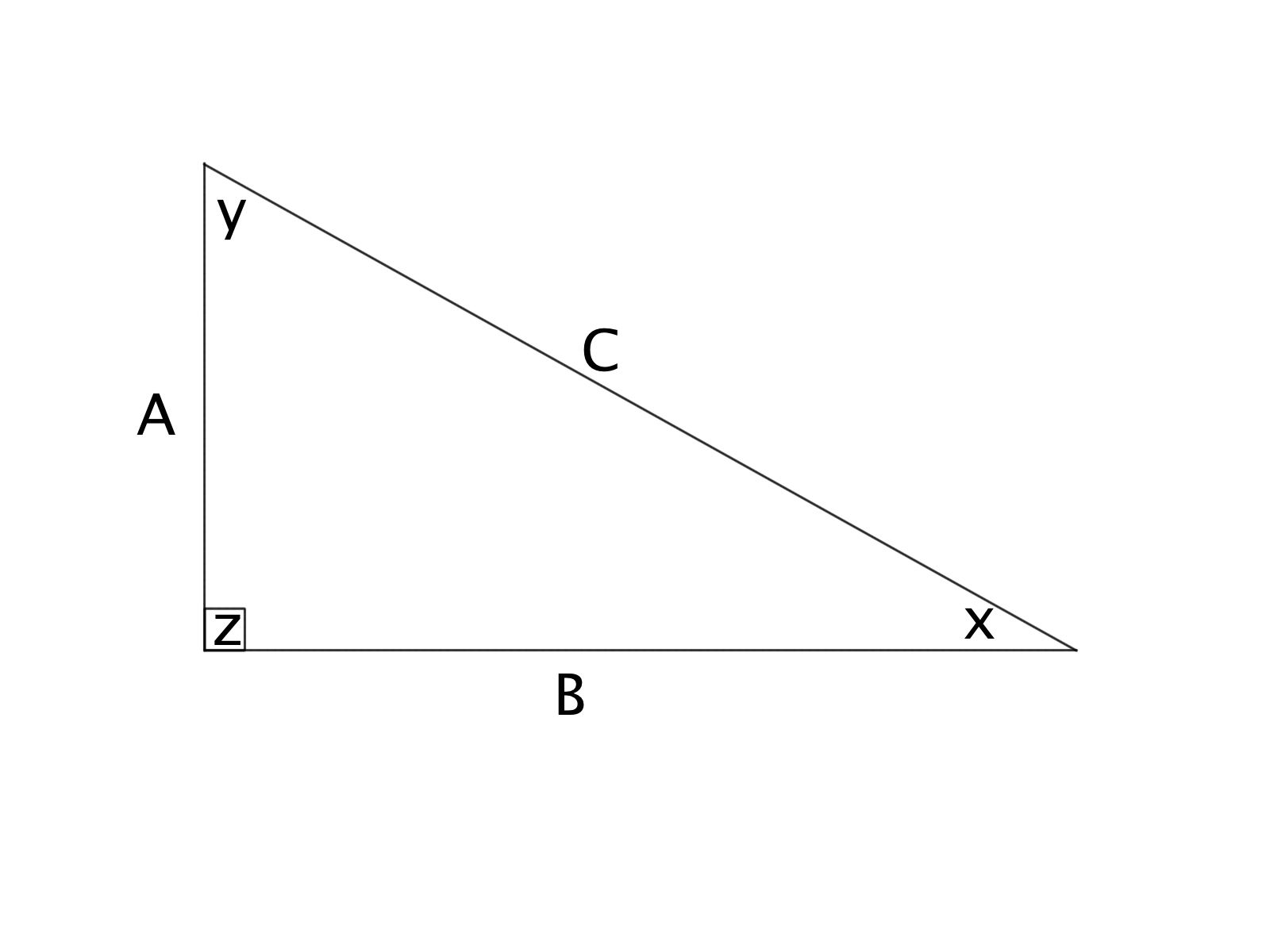Triangle ABC is a right triangle. If the length of side A = 3 inches and C = 5 inches, what is the length of side B?

6 inches

4 inches

1 inches

1/2 inches

4.5 inches

4 inches

Explanation:

Using the Pythagorean Theorem, we know that.

This gives: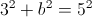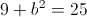Subtracting 9 from both sides of the equation gives: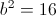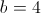inchesExample Question #23 : How To Find The Length Of The Hypotenuse Of A Right Triangle : Pythagorean TheoremTriangle ABC is a right triangle. If the length of side A = 8 inches and B = 11 inches, find the length of the hypoteneuse (to the nearest tenth).

185 inches

14.2 inches

13.7 inches

13.6 inches

184 inches

13.6 inches

Explanation:

Using the Pythagrean Theorem, we know that.

This tells us: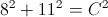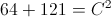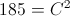Taking the square root of both sides, we find that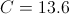inches

Example Question #21 : How To Find The Length Of The Hypotenuse Of A Right Triangle : Pythagorean TheoremGiven:

A = 6 feet

B = 9 feet

What is the length of the hypoteneuse of the triangle (to the nearest tenth)?

10.6 feet

10.2 feet

10.8 feet

10.5 feet

10.1 feet

10.8 feet

Explanation:

Using the Pythagrean Theorem, we know that.

This tells us: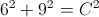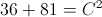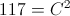Taking the square root of both sides, we find that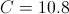Example Question #25 : How To Find The Length Of The Hypotenuse Of A Right Triangle : Pythagorean TheoremGiven:

A = 2 miles

B = 3 miles

What is the length of the hypoteneuse of triangle ABC, to the nearest tenth?

3.4 miles

3.2 miles

3.5 miles

3.7 miles

3.6 miles

3.6 miles

Explanation:

Using the Pythagrean Theorem, we know that.

This tells us: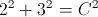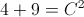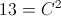Taking the square root of both sides, we find that